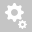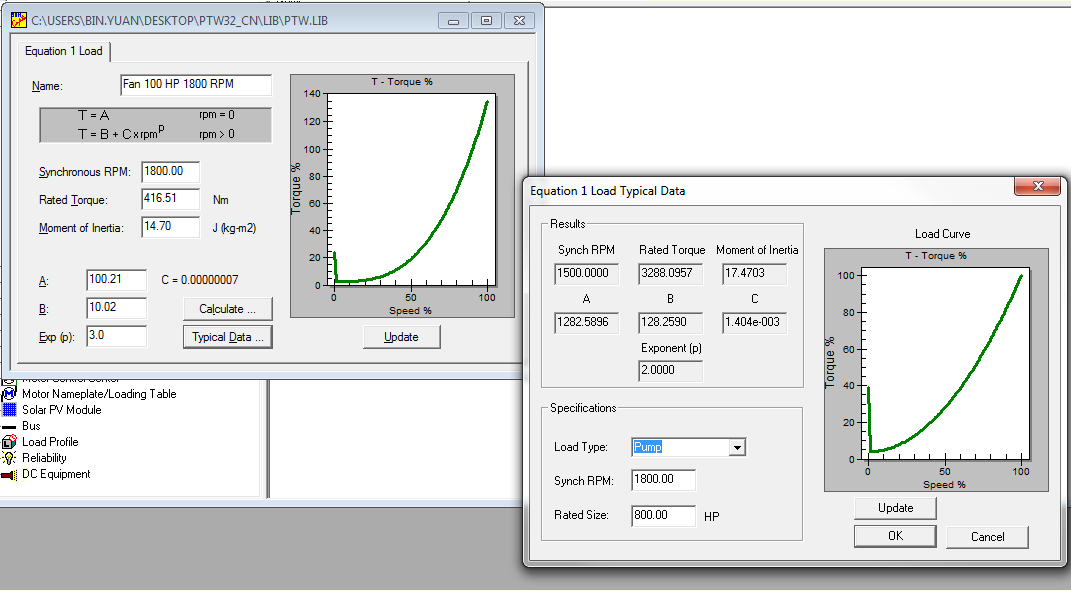##Recently viewed tickets• Sales
• Library Model Request
• Technical Support

# Formula used to calculate typical load exponential data

1. Based on the load type, a breakaway torque factor and exponent p are assigned:
Pump: 0.4, p=2
Motor_Gen_Set: 0.25,p=2
Blower: 0.3,p=3
Grinder: 0.3,p=3
Compressor: 0.4,p=2
Fan: 0.25,p=3
Other: 0.4 ,p=2
2. A is calculated by HP*5252/speed/.987*breakawayfactor
3. B is calculated by A*0.1
4. C is calculated by (by HP*5252/speed/.987 –B) /(0.987*speed)^p
5. Formula used to convert Ftlbs to NM:
6. NM=ftlbs/0.7376372